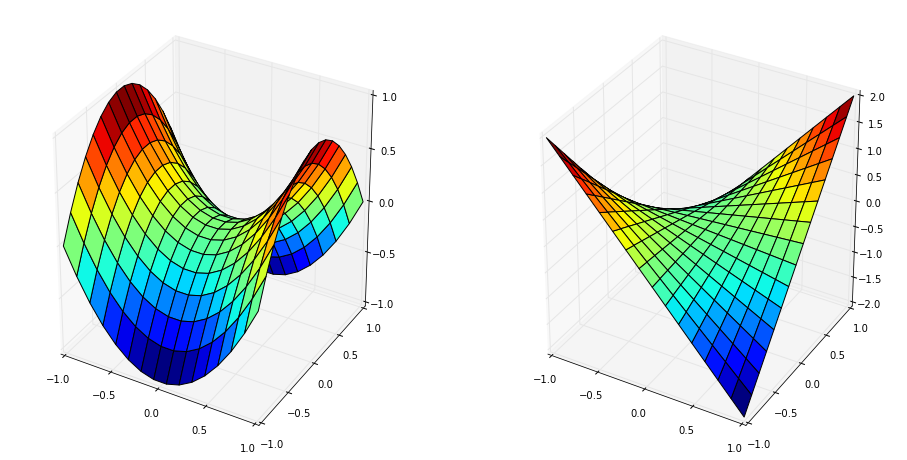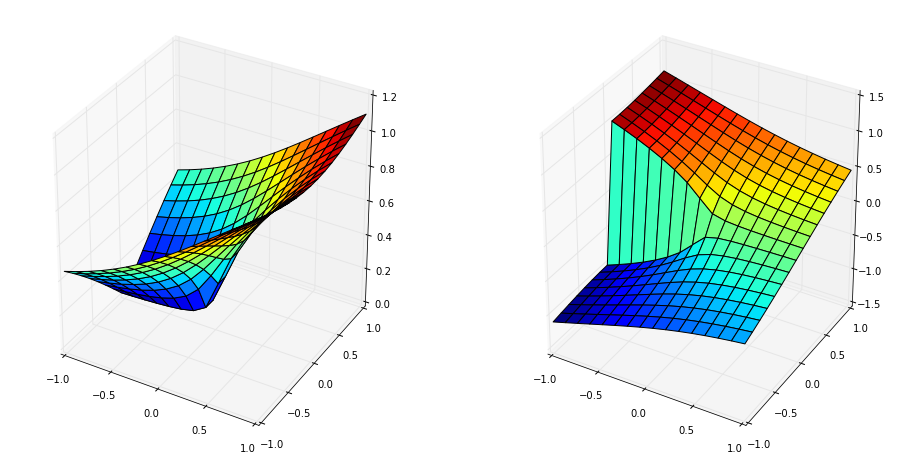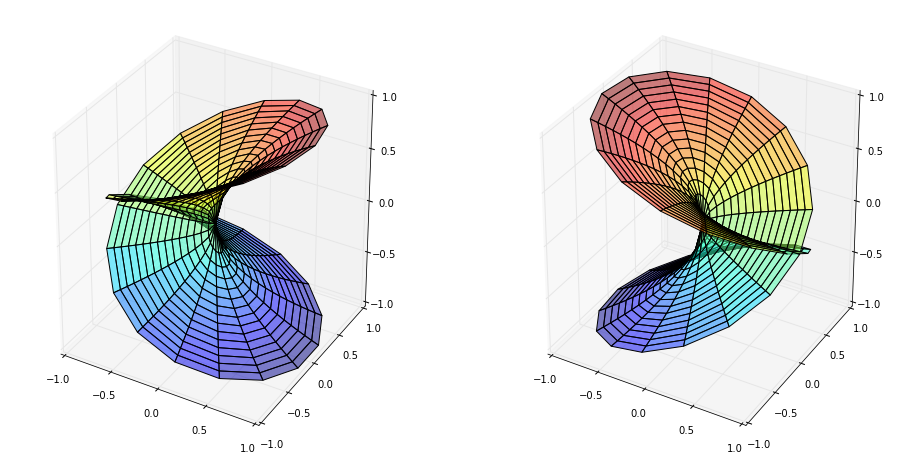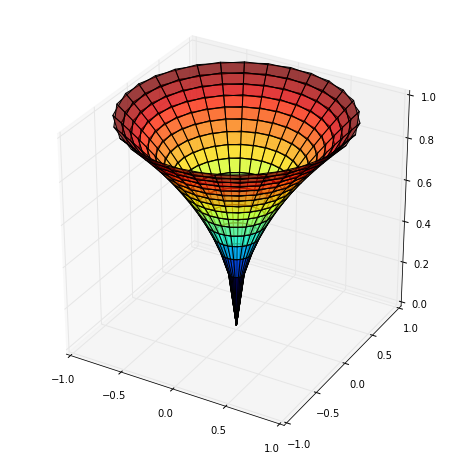In :
%matplotlib inline

In :
import numpy
import sympy

from mpl_toolkits.mplot3d import Axes3D
import matplotlib
from matplotlib import cm
from matplotlib import pyplot as plt


A Naive Approach¶

...consisting of creating a complex grid and simply taking the square root of the grid.

In :
a,b = -1,1
n = 16
x,y = numpy.mgrid[a:b:(1j*n),a:b:(1j*n)]
z = x + 1j*y
w = z**2

In :
fig = plt.figure(figsize=(16,8))

# plot the real part
ax_real.plot_surface(z.real, z.imag, w.real,
rstride=1, cstride=1, cmap=cm.jet)

# plot the imaginary part
ax_imag.plot_surface(z.real, z.imag, w.imag,
rstride=1, cstride=1, cmap=cm.jet)

Out:
<mpl_toolkits.mplot3d.art3d.Poly3DCollection at 0x43ccf10>In :
a,b = -1,1
n = 16
x,y = numpy.mgrid[a:b:(1j*n),a:b:(1j*n)]
z = x + 1j*y
w = numpy.sqrt(z)

In :
fig = plt.figure(figsize=(16,8))

# plot the real part
ax_real.plot_surface(z.real, z.imag, w.real,
rstride=1, cstride=1, cmap=cm.jet)

# plot the imaginary part
ax_imag.plot_surface(z.real, z.imag, w.imag,
rstride=1, cstride=1, cmap=cm.jet)

Out:
<mpl_toolkits.mplot3d.art3d.Poly3DCollection at 0x43dbcd0>The Issue¶

In many (all) complex square-root functions a branch cut is chosen (usually the negative real axis) and only one branch is computed in order to make square root single-valued.

From complex analysis we learn that we can use polar coordinates to easily verify that $w = \sqrt{z}$, which I will read as $w^2 = z$ since the latter makes it clear that I won't be chosing a branch of $w$ / making a branch cut in the $z$ plane. Because the branch point $z=0$ is 2-ramified / has branching number two I will need to make two rotations to capture all of the behavior near the branch point.

In :
branching_number = 2

Nr = 16
Ntheta = 32

# compute the theta,R domain
theta = numpy.linspace(0,2*numpy.pi*branching_number, Ntheta)
r = numpy.linspace(0,1,Nr)
Theta, R = numpy.meshgrid(theta,r)

z = R*numpy.exp(1j*Theta)

# compute w^2 = z. THE KEY IDEA is to pass the exponentiation by 1/2 into exp().
w = numpy.sqrt(R)*numpy.exp(1j*Theta/2)

In :
fig = plt.figure(figsize=(16,8))

# plot the real part
ax_real.plot_surface(z.real, z.imag, w.real,
rstride=1, cstride=1, cmap=cm.jet, alpha=0.5)

# plot the imaginary part
ax_imag.plot_surface(z.real, z.imag, w.imag,
rstride=1, cstride=1, cmap=cm.jet, alpha=0.5)

Out:
<mpl_toolkits.mplot3d.art3d.Poly3DCollection at 0x4a3d110>In :
# plot abs(w)
fig = plt.figure(figsize=(8,8))

<mpl_toolkits.mplot3d.art3d.Poly3DCollection at 0x45f36d0>